Maple package NOPH 1.0

Based on Wu’s elimination method, the homotopy analysis method as well as Padé technique, a Maple package NOPH 1.0 is developed, which can automatically deliver accurate approximate solutions of nonlinear oscillation system with the form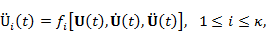subject to initial conditions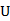k(0)=ak +dk,(0)=0,i(0)=ai+di,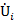(0)=wbi,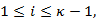where the dependent variable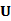(t)=(1(t), ¼ ,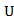k(t)), the mean of motion d=(d1, ¼ ,dk), w denotes the frequency of oscillation, the independent variable t denotes time, the dot denotes differentiation with respect to t,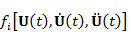is an algebraic or rational function ofand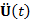.

As an open resource, the NOPH 1.0 can be free downloaded at the following hyperlinks. The higher version of the NOPH will be issued in future.

Source Code:    Zip form of NOPH 1.0 (for Maple 15)